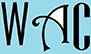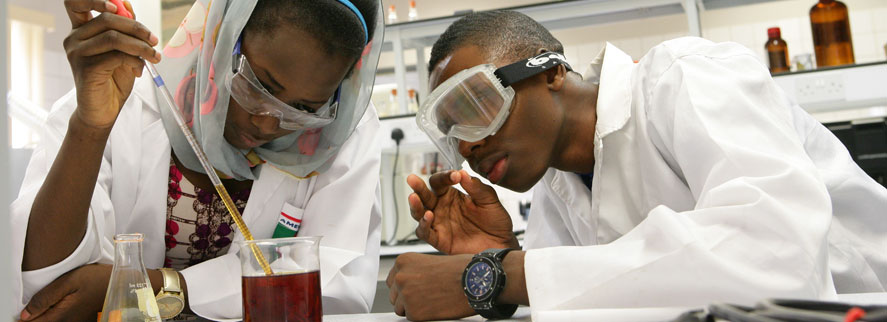## Algebra Question for Title 1 Schools: Discount Question?

11) A 20% discount saves Pat \$45 for a chair.  How much did he pay for the chair?

20% = 20/100 = 0.2

x = original price

x – 0.2x = x – 45 (subtract the 20% discount, which is equivalent to \$45, from the original price)

you have to make “x” subject of formula by moving all the “x” to one side. When you go to the other side, addition becomes subtraction, multiplication becomes division, and vice versa.

x – x – 0.2x = -45

-0.2x = -45

x = -45/-0.2 (-0.2 was multiplication at the other end)

x = \$225 original price

225 – 45 = \$180 was paid for the chair